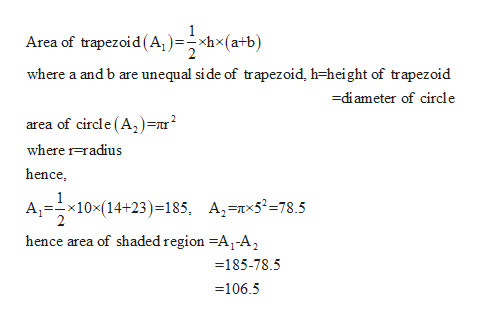# 23 in.5 in14 in.

Question

check_circle

Step 1

Given figure is a circle with outside trapezoid

From figu...help_outlineImage TranscriptioncloseArea of trapezoid(A,)=hx(a+b) where a and b are unequal side of trapezoid, h=height of trapezoid -diameter of circle area of circle (A,)=nr2 where rradius hence, 10x(14+23) = 185, A,=nx5*=78.5 hence area of shaded region =A,-A, 185-78.5 =106.5 fullscreen

### Want to see the full answer?

See Solution

#### Want to see this answer and more?

Solutions are written by subject experts who are available 24/7. Questions are typically answered within 1 hour.*

See Solution
*Response times may vary by subject and question.
Tagged in

### Geometry